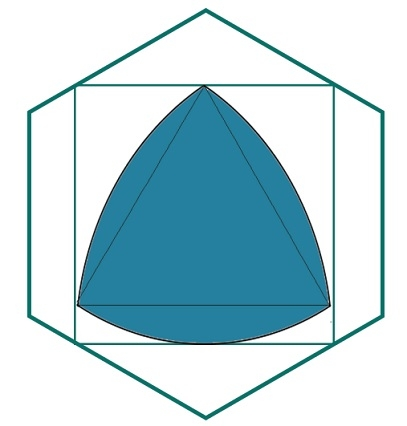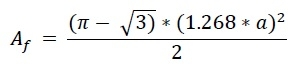# Biggest Reuleaux Triangle inscribed within a square which is inscribed within a hexagon?

Here we will see the area of biggest Reuleax triangle inscribed within a square which is inscribed in a regular hexagon. Suppose ‘a’ is the side of the hexagon. The side of the square is x and the height of the Reuleaux triangle is h.From the formula of each side of inscribed square inside one hexagon is −

𝑥 = 1.268𝑎

The height of the Reuleaux triangle is the same as x. So x = h. So the area of the Reuleaux triangle is −## Example

#include <iostream>
#include <cmath>
using namespace std;
float areaReuleaux(float a) { //side of hexagon is a
if (a < 0) //if a is negative it is invalid
return -1;
float area = ((3.1415 - sqrt(3)) * (1.268*a) * (1.268*a))/2;
return area;
}
int main() {
float side = 5;
cout << "Area of Reuleaux Triangle: " << areaReuleaux(side);
}

## Output

Area of Reuleaux Triangle: 28.3268